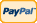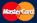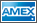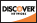Online Store Software Catalog and Price Product Registration Services News About Us Contact Us My Account Español
•Electronics, electrical engineering
•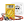Engineering physics
•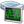Generators
•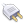Interfaces
•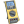Multimeters
•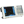Oscilloscopes
•Persona
•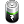Power supplies
•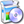Software
•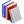Standards
•Thesaurus
•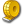Units of measurement
Filter by first letter
A
B
C
D
E
F
G
H
I
J
K
L
M
N
O
P
Q
R
S
T
U
V
W
X
Y
Z
+
-

# Celsius (°C)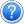Search: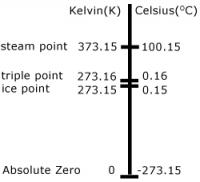Celsius, also known as centigrade, is a scale and unit of measurement for temperature. It is named after the Swedish astronomer Anders Celsius, who developed a similar temperature scale. The degree Celsius (°C) can refer to a specific temperature on the Celsius scale as well as a unit to indicate a temperature interval, a difference between two temperatures or an uncertainty. The unit was known until 1948 as "centigrade" from the Latin centum translated as 100 and gradus translated as "steps".

From 1744 until 1954, 0°C was defined as the freezing point of water and 100°C was defined as the boiling point of water, both at a pressure of one standard atmosphere with mercury being the working material. At first a temperature scale was the reverse of the scale now known by the name "Celsius": 0 represented the boiling point of water, while 100 represented the freezing point of water. But in 1744, coincident with the death of Anders Celsius, the Swedish botanist Carolus Linnaeus (1707–1778) reversed Celsius's scale upon receipt of his first thermometer featuring a scale where zero represented the melting point of ice and 100 represented the boiling point.

By international agreement the unit "degree Celsius" and the Celsius scale are currently defined by two different temperatures: absolute zero, and the triple point of VSMOW (specially purified water). This definition also precisely relates the Celsius scale to the Kelvin scale, which defines the SI base unit of thermodynamic temperature with symbol K. Absolute zero, the lowest temperature possible at which matter reaches minimum entropy, is defined as being precisely 0 K and −273.15 °C. The temperature of the triple point of water is defined as precisely 273.16 K and 0.01 °C.

This definition fixes the magnitude of both the degree Celsius and the kelvin as precisely 1 part in 273.16 (approximately 0.00366) of the difference between absolute zero and the triple point of water. Thus, it sets the magnitude of one degree Celsius and that of one kelvin as exactly the same. Additionally, it establishes the difference between the two scales' null points as being precisely 273.15 degrees Celsius (−273.15 °C = 0 K and 0 °C = 273.15 K).

Back to the list

Units Converter
• How it Works
• Company News
• Industry News & Events
• Measurement Fun Facts